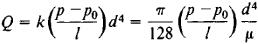# Poiseuille's Law

Also found in: Dictionary, Thesaurus, Medical, Legal, Wikipedia.
Related to Poiseuille's Law: Poiseuille's equation

## Poiseuille's law

[pwä′zə·ēz ‚lȯ]
(fluid mechanics)
The law that the volume flow of an incompressible fluid through a circular tube is equal to π/8 times the pressure differences between the ends of the tube, times the fourth power of the tube's radius divided by the product of the tube's length and the dynamic viscosity of the fluid.

## Poiseuille’s Law

the law governing the flow of a liquid through a thin cylindrical tube. It states that the volume Q per second of a liquid flowing through a cross section of the tube is directly proportional to the pressure difference between p and p0 at the entrance of the tube and at the outlet and to the fourth power of the diameter d of the tube and inversely proportional to the length l of the tube and the viscosity μ of the liquid:This formula was derived by J. L. M. Poiseuille, and the relation of the coefficient k to the viscosity μ was established later by G. Stokes: k = π/(128μ).

Poiseuille’s law is applicable only to a liquid in laminar flow (in practice, for very thin tubes) and on the condition that the length of the tube greatly exceed the length of the initial section, in which the laminar flow develops in the tube. Poiseuille’s law is used in determining the viscosity of liquids at different temperatures by means of capillary viscometers.

Mentioned in ?
References in periodicals archive ?
As is was shown by direct experimental measurements of the pressure-flow relationships in separate vessels, veins, and vein systems, the hydraulic conductivity of the plant conducting pathways is described by Poiseuille's law [delta]P = QZ, where Z = a[Z.
Poiseuille's law describes fluid flow in a circular pipe as: Q = [pi][delta]P[r.
Principle of operation is based on Poiseuille's Law of capillary flow, which states that the pressure drop of a fluid flowing though a capillary is directly proportional to the viscosity.

Site: Follow: Share:
Open / Close